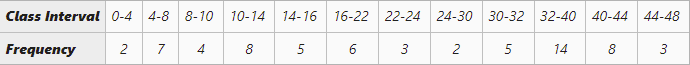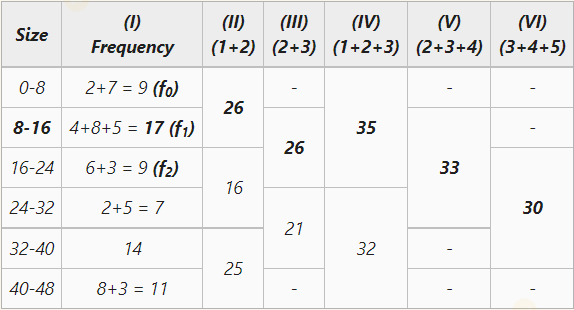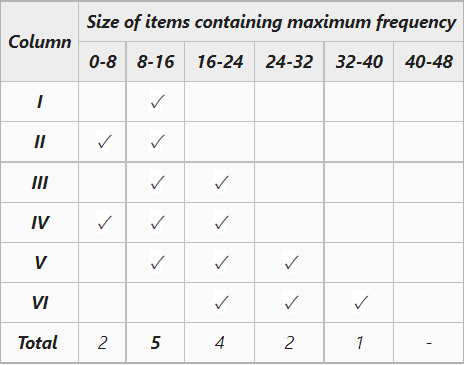GFG App
Open AppBrowser
Continue

# Calculation of Mode in Special Cases

The word mode is derived from the French word ‘La Mode’, meaning anything that is in fashion or vogue. A measure of central tendency in statistical series that determines the value occurring most frequently in the given series is known as mode. In other words, the modal value of the series has the highest frequency in the given series. For example, if in a class of 100 students, 20 students have opted for Mathematics, 50 students have opted for IP, and the rest 30 have opted for Physical Education, then the modal opted subject of the class would be IP.

Mode is represented by the letter Z and can be calculated in three different series; viz., individual series, discrete series, and continuous series.

According to Kenny, “The value of the variable which occurs most frequently in a distribution is called the mode.”

According to Croxton and Cowden, “The mode may be regarded as the most typical of a series of values.”

## Calculation of Mode in Special Cases

### 1. Mode, when Class Intervals are Unequal

In the case of unequal class intervals, the mode is determined by making the class intervals equal. After that, the mode is determined through the usual process using the inspection or grouping method.

#### Example:

Calculate mode of the following series.#### Solution:Grouping TableAnalysis Table

It is clear from the Analysis Table that the modal class interval is 8-16.

The formula for calculating Mode is,In the given series,= 8 + 10.67

= 18.67

The mode of the given series is 18.67

### 2. Mode in Inclusive Series

In an inclusive series, the upper limit of the class interval is not the same as the lower limit of the next class interval. To determine the mode of an inclusive series, first of all, it is converted into an exclusive series. It means that the upper limit of the class interval and the lower limit of the next class interval are made equal.

#### Conversion of Inclusive Series into Exclusive Series

For statistical calculation, sometimes it becomes necessary to convert the inclusive series into exclusive series. Suppose, in the above example, some students have obtained marks such as 10.5, 40,5, etc. In this case, this series will be converted into exclusive series.

The steps for converting an inclusive series into exclusive series are:

• In this first step, calculate the difference between the upper class limit of one class interval and the lower limit of the next class interval.
• The next step is to divide the difference by two, and then add the resulting value to the upper limit of every class interval and subtract it from the lower limit of every class interval.

#### Example:

Calculate mode of the following series.

#### Solution:

The formula for calculating Mode is,28 is the highest frequency, which means that the modal class interval is 24.5-29.5

In the given series,= 24.5 + 2.4

= 26.9

The mode of the given inclusive series is 26.9

### 3. When only Mid-Values of the Class Intervals are given

In the case of a series with mid-values of the Class Intervals, it is first expanded as a series with class intervals or simple frequency distribution series. After that, the mode is determined using the usual practice through Inspection or Grouping Method.

#### Conversion of Mid-Value Frequency Series into Simple Frequency Series

The steps to convert a mid-value frequency series into a simple frequency series are as follows:

• The first step is to determine the mutual difference between the mid-values.
• The next step is to obtain half of the resulting difference.
• The last step of conversion is to subtract the resulting figure from the second step from the mid-value to get the lower limit of the class interval, and add the resulting figure from the second step to the mid-value to get the upper limit.m = Mid-Values

i = Difference between mid-values#### Example:

Calculate the mode of the following series.

#### Solution:

25 is the highest frequency, which means that the modal class of the series is 20-30.

The formula for calculating Mode is,In the given series,= 20 + 3.75

= 23.75

The mode of the given series is 23.75

### 4. Mode in ‘Less than’ Cumulative Frequency Distribution

In the case of the ‘Less than’ Cumulative Frequency Distribution series, it is first converted into a normal frequency distribution series, and then the mode is determined through the usual process using the Inspection or Grouping Method.

#### Example:

Calculate the mode of the following ‘Less than’ Cumulative Frequency Distribution Series.

#### Solution:

14 is the highest frequency, which means that the modal class of the series is 3000-4000.

The formula for calculating Mode is,In the given series,= 3000 + 692.30

= 3692.30

The modal income of the given series is ₹3692.30

### 5. Mode through Arithmetic Mean and Median

There is a relationship between Mode, Arithmetic Mean, and Median through which any of the measures of central tendency can be determined if the rest of the two are given. The formula for calculating mode through the relationship is,Z is Mode, M is Median, andThis formula is usually used in the normal or symmetrical distribution, as the mean, median, and mode coincide in this case. However, the formula can also be used in an asymmetrical distribution.

#### Example:

Calculate the mode of a series with Mean and Median as 24 and 30, respectively.

#### Solution:

Mode = 3 Median – 2 Mean= 3(30) – 2(24)

= 90 – 48

= 42

The mode of the given series is 42.

My Personal Notes arrow_drop_up